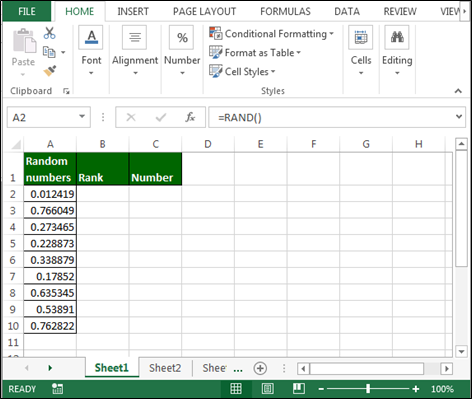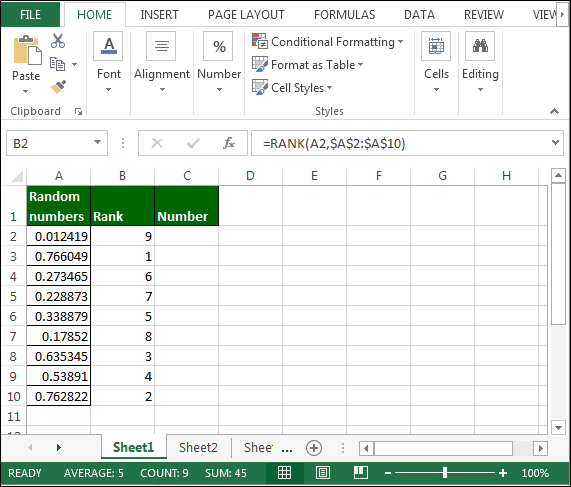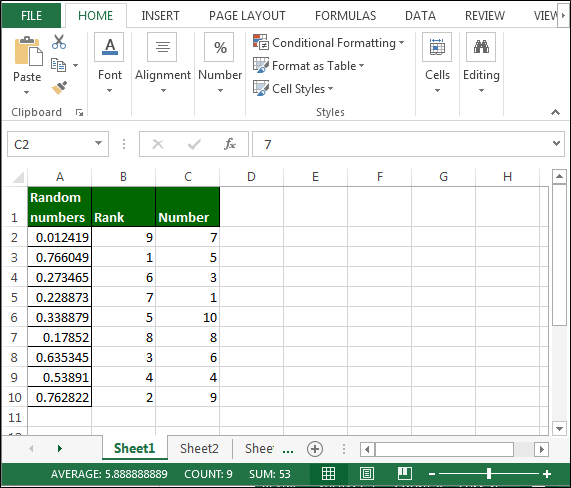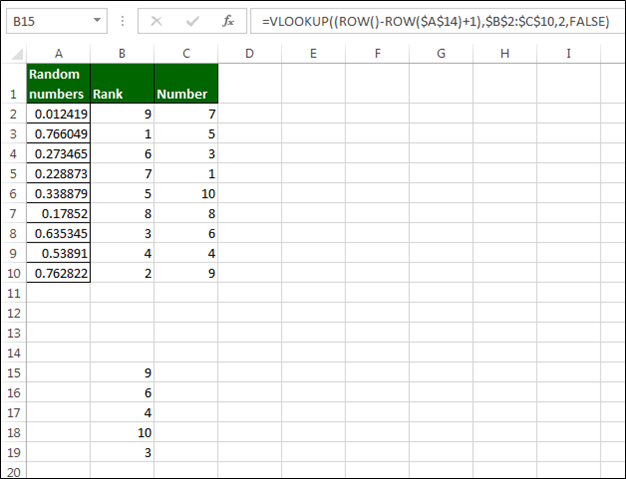# Selecting a Set of Random Numbers, Avoiding Duplicates

In this article, we will learn how and where we can generate random numbers without repetition in Microsoft Excel.

We need the random numbers when we want to choose the lottery numbers. We need lottery numbers at so many places for admission, savings, etc.

To return the random numbers, avoiding duplicates, we will use Rand, Rank and VLOOKUP function along with row function. We use Rand function to select a set of 5 random numbers between 1 and 10, without getting duplicates.

Let’s take an example to understand.

We want to return lucky lottery numbers. So, to generate the lucky numbers, firstly, we will generate random numbers by using Rand function.

In data range A1:C11, Step 1:-

• Enter Rand function in Column A :- = RAND()Note: - When we will do any activity in the sheet, every time rand function will change the random numbers.

Now, we will return the rank to every random number’s by using the Rank function.

• Enter the following formula in Column B:- =RANK(A2,\$A\$2:\$A\$10)• Enter the numbers 1-10 in column C.• In lottery range (A14:B19), in column B, use the VLOOKUP and ROW functions in the following formula:-
• =VLOOKUP((ROW()-ROW(\$A\$19)+1),\$B\$2:\$C\$12,2,FALSE)As a result, 5 unique random numbers from 1 to 10 will be displayed in Column B. The numbers will change with each recalculation.

This is the way we can select a set of random numbers avoiding duplicates by using the formulae in Microsoft Excel.1.